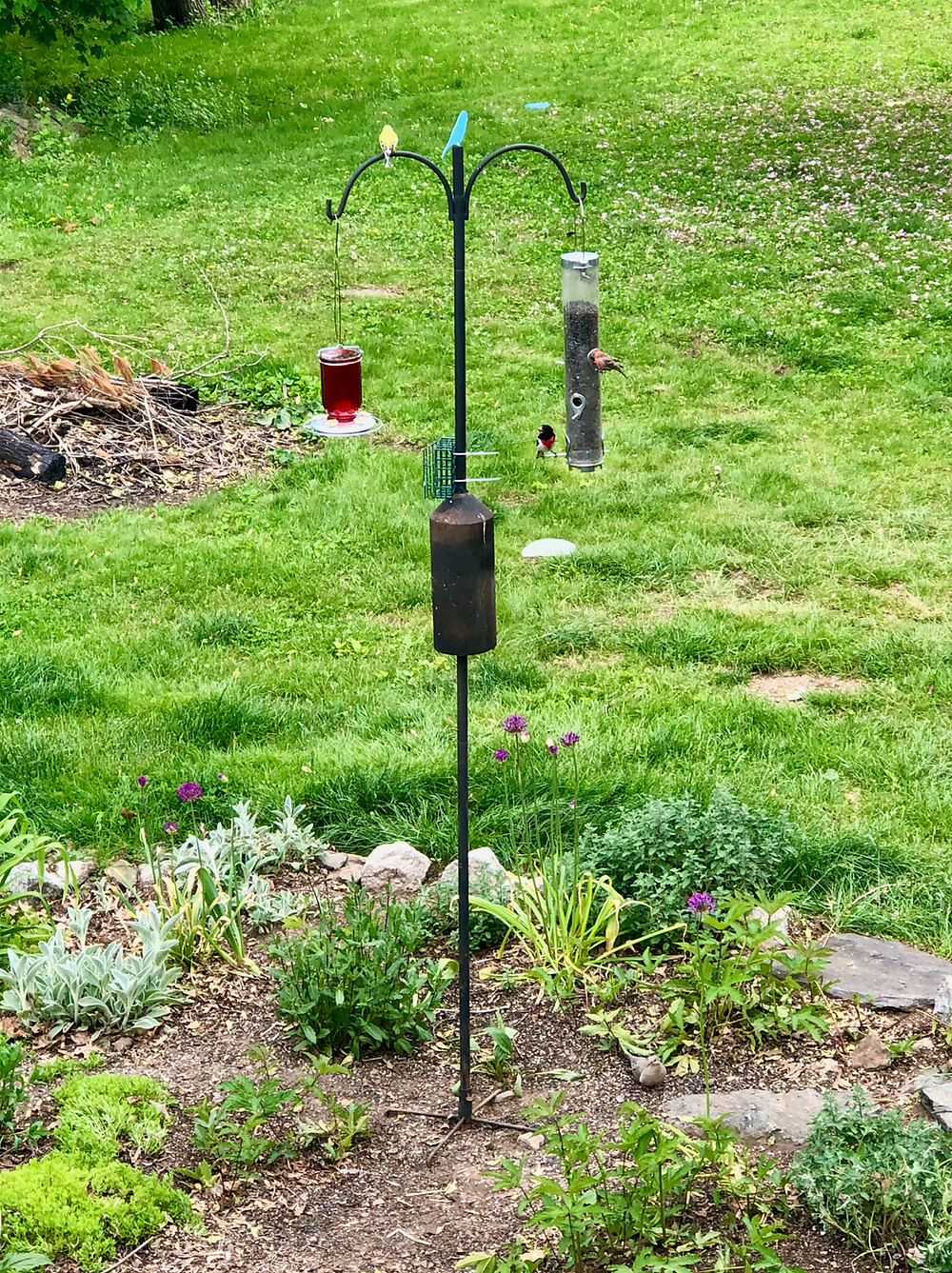Search
•Tim Burns

# An ML model for Birds in my Back YardI feel like I am hunting and pecking my way into Machine Learning. Get it? Birding... pecking... Sorry, I'm just a loser dad.

I find myself looking for data that will lend itself to Logistic Regression. What kinds of binary classification can you do with birding data?

### Male or Female?

The goldfinch and the cardinal both are sexually dichromatic, meaning that the male and the female differ in color. A good candidate for a Machine Learning toy algorithm is one that can guess the sex of a bird based on its color.

The Logistic Regression will learn with color and guess the sex based on color. Here is the Matplotlib code to illustrate the function used in Logistic Regression.

```#%%
import numpy as np
import math

sigmoid_y = []
range_x = np.arange(-10, 10, 0.1)
for val_x in range_x:
sigmoid_y.append(1.0 / (1.0 + math.exp(-val_x)))

import matplotlib.pyplot as plt

fig, axes = plt.subplots(1, 1)
set_colors(axes)

axes.set_title('The Sigmoid Function', color='white')
axes.plot(range_x, sigmoid_y)

```